Examples
We demonstrate ARDL and NARDL estimation using a dataset from Greene (2008, page 685). This dataset consists of a number of quarterly US macroeconomic variables between 1950 and 2000. The data are in the workfile “Ardl13.WF1”
Example 1: Symmetric ARDL (Automatic Lag Selection)
We start with a classical (symmetric) ARDL model using the log of real consumption as the dependent variable, and the log of real GDP as a single regressor (along with a constant). Bring up the estimation dialog and enter
log(realcons) log(realgdp)
into the Linear dynamic specification edit field and select Automatic selection with a maximum of 8 lags (two years) for both the dependent variable and dynamic regressors.
We include a full set of quarterly dummies as fixed regressors. In particular, add a restricted constant to the cointegrating relationship, and the remaining quarterly dummies to the short-run regressors. Include the long-run constant by choosing Rest. Constant in the Trend specification dropdown menu, and enter
@expand(@quarter, @droplast)
into the Fixed regressors edit field to add the remaining dummy variables.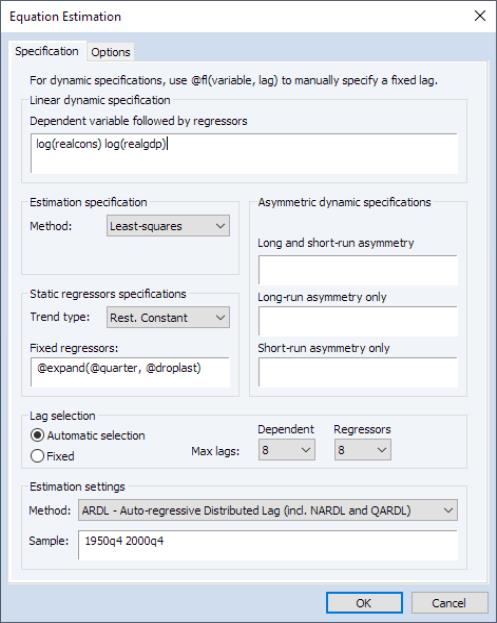We do not make changes in the Options tab, leaving all settings at their default value. The estimation results for this specification are shown below: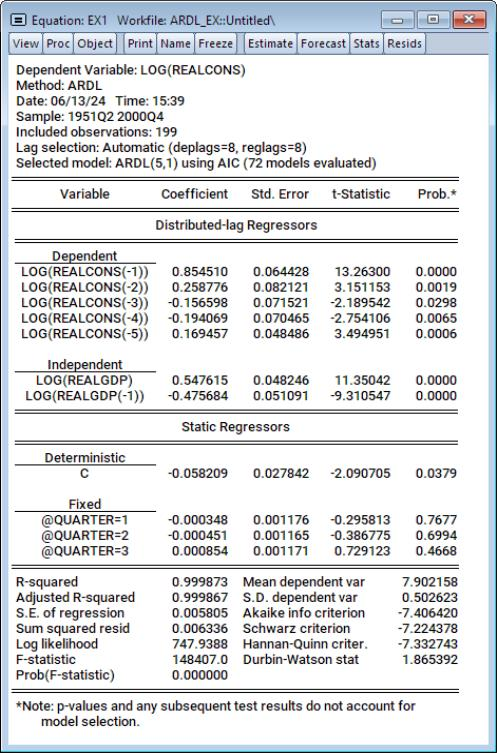The first part of the output gives a summary of the settings used during estimation. Here we see that lags were determined using automatic selection (Akaike Information Criterion) with a maximum of 8 lags of both the dependent variable and the regressor. Out of the 72 models evaluated, the automatic selection procedure yielded an ARDL(5,1) model—5 lags of the dependent variable LOG(REALCONS), and a single lag (along with the level value) of the regressor LOG(REALGDP).
The rest of the output is standard least squares output for the selected model. Note that each of the regressors (with the exception of the quarterly dummies) is statistically significant, and that the coefficient on the one period lag of the dependent variable, LOG(REALCONS), is quite high, at 0.85.
To view the performance of the selected model against the alternatives, we click on \View/Model Selection Summary/Criteria Graph to view a graph of the AIC of the top twenty models: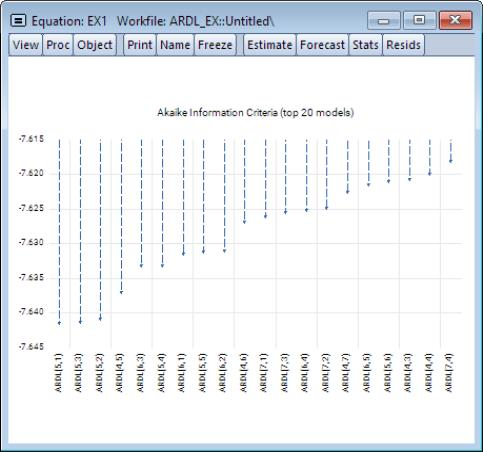The selected ARDL(5,1) model was only slightly better than an ARDL(5,2) model, which was in turn only slightly better than an ARDL(5,3). Note that the top three models all employ five lags of the dependent variable.
Click on the Name button on the equation toolbar and name the equation EX1.
Example 2: Symmetric ARDL(3,3)
Instead of using automatic selection to choose the best model, Greene (Example 20.4) analyzes these data with a fixed ARDL(3,3) model. We can estimate this specification by clicking on Object/Copy on the EX1 toolbar to make a copy of the existing equation, name the equation EX2, then pressing the Estimate button to bring up the estimation dialog. Next, change the number of lags on both dependent and regressors to “3”, and then select the Fixed radio button to switch off automatic lag selection: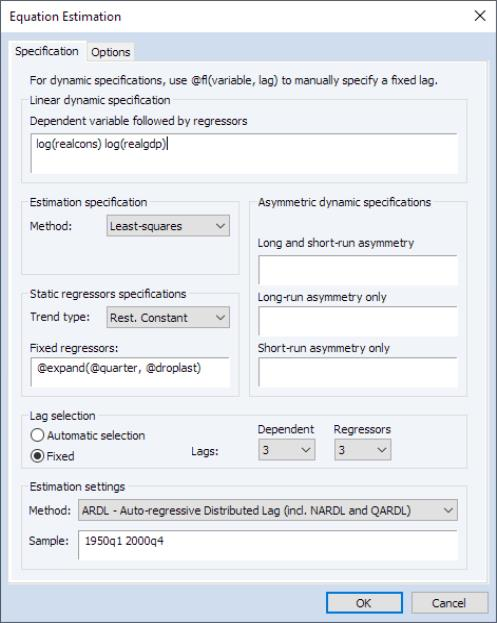Click on OK to estimate the equation using these settings.
The results of this estimation are given by: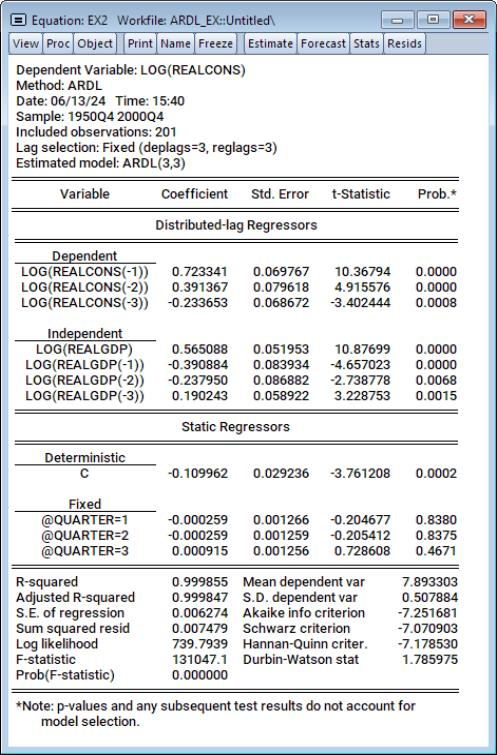For this specification, the one-period lag on the dependent variable remains high, at 0.72, and again all coefficients are statistically significant (with the exception of the period dummies).
We may examine the CEC and EC forms of the estimates by selecting View/ARDL Diagnostics/Error Correction Results. EViews will display long-run output in the form of a spool with two tables showing the Conditional Error Correction regression results, and the Error Correction results. The first table displays the estimation results in the CEC form: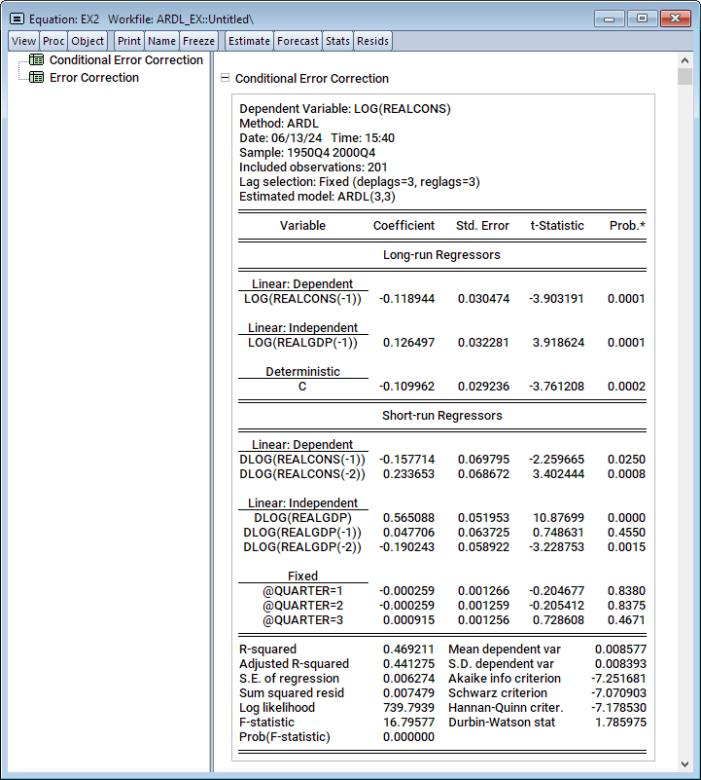The EC results, which are displayed click on the Error Correction node, show that the speed of adjustment coefficient is negative (-11.89) and statistically significant.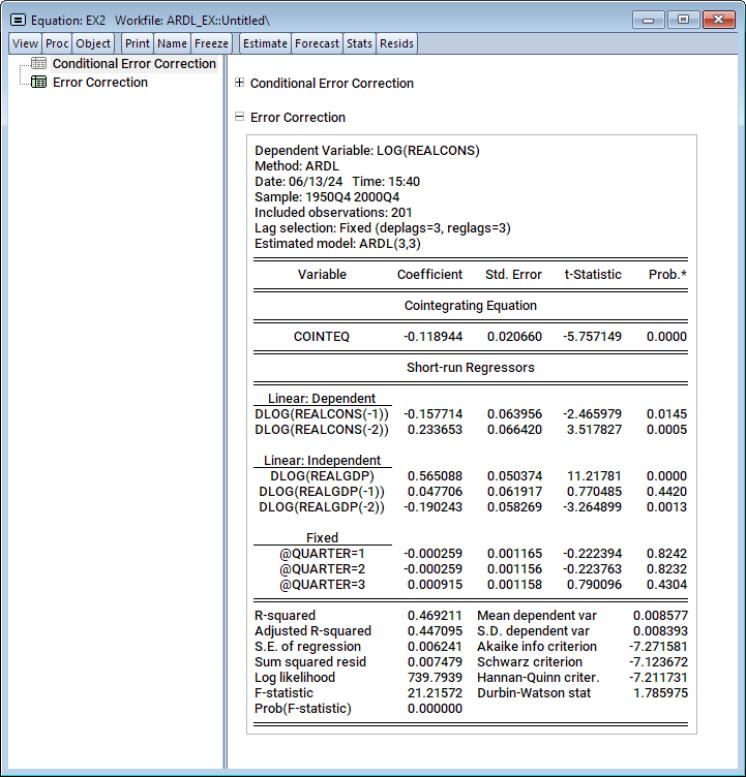Clicking on View/ARDL Diagnostics/Cointegrating Relations shows the specification and coefficient results for the cointegrating relationship: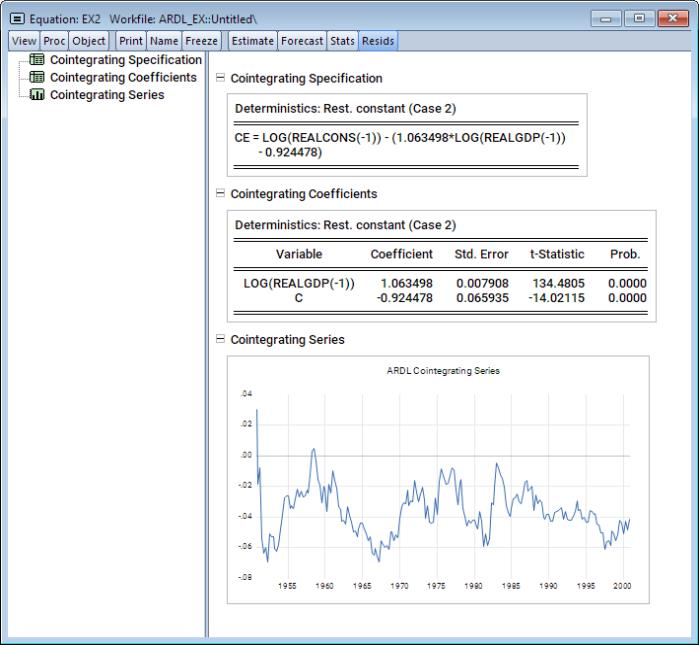The equilibrium coefficients show that the impact of a change in LOG(REALGDP) on LOG(REALCONS) is close to unity. Moreover, since the specification restricts the constant to the long-run, it shows up as part of the cointegrating equation.
Example 3: Symmetric ARDL(1,1)
As a final symmetric ARDL example, we will consider Greene's Example 20.5 which estimates an ARDL(1,1) model. Copy the EX2 ARDL equation object, name the copy EX3, bring up the estimation dialog by clicking on the Estimate button and change the number of lags to “1” for both dependent and regressors, remove the quarterly dummies, and then click on OK to estimate with the new specification: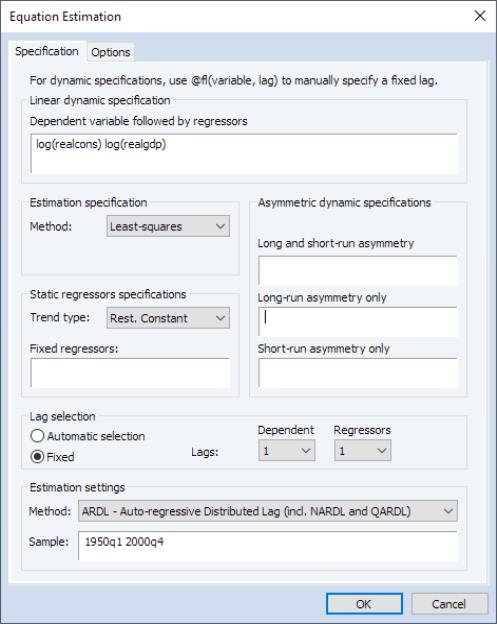The results obtained from estimating this specification are given by: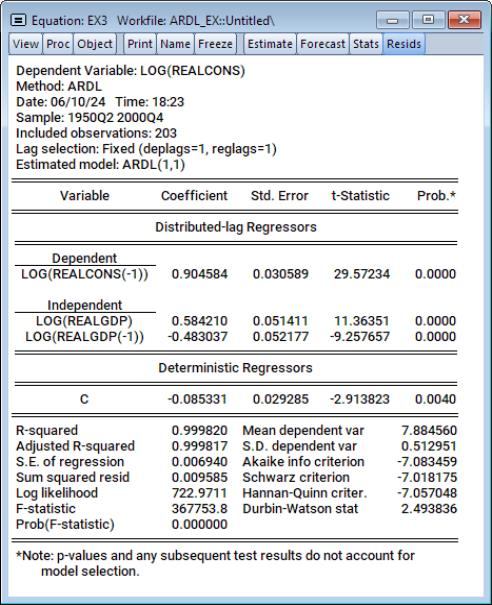Following estimation, we may perform bounds test for cointegration by clicking on View/ARDL Diagnostics/Bounds Test to bring up the cointegrating relationship view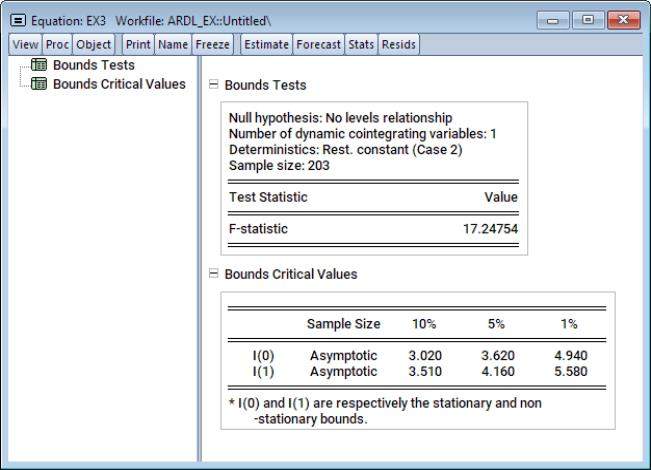The bounds statistic value is 17.25. We compare this result to the critical values listed in the second table. Clearly the statistic is larger than the I(1) critical value at all significance levels so that we may reject the null hypothesis of no levels relationship and conclude that LOG(REALGDP) and LOG(REALCONS) are cointegrated.
We may also evaluate the cumulative dynamic multiplier of the explanatory variable LOG(REALGDP) on LOG(REALCONS). Click on View/ARDL Diagnostics/Dynamic Multiplier Graph... to bring up the dynamic multiplier dialog: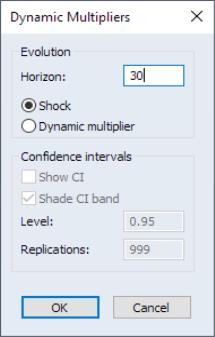Note that confidence interval settings are not available since we have estimated a purely symmetric ARDL models. Enter “30” as the horizon length and click on OK.
EViews will display a spool object with a dynamic multiplier graph for each distributed lag variable: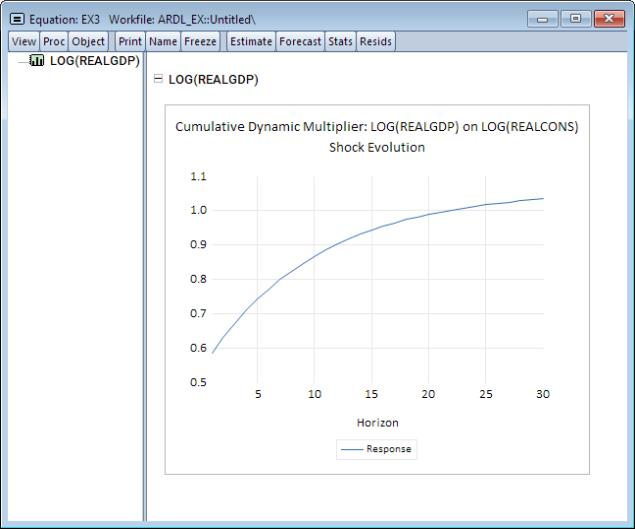Here we see that LOG(REALGDP) approaches its long-run value of 1.06 as the horizon length increases. Moreover, it does so at a diminishing pace.
Example 4: Asymmetric ARDL(1,1,1)
Here we will continue from the previous example, but consider the NARDL(1,1,1) model of LOG(REALCONS) on LOG(REALGDP) and LOG(REALGOVT). We will treat LOG(REALGOVT) as an asymmetric variable which is asymmetric in both the short-run and the long-run.
To estimate this model, copy the EX3 equation, name the copy EX4, then bring up the estimation dialog by clicking on the Estimate button. Next, add an Asymmetric dynamic specification group by entering “LOG(REAGOVT)” in the Long-run and short-run asymmetry edit field,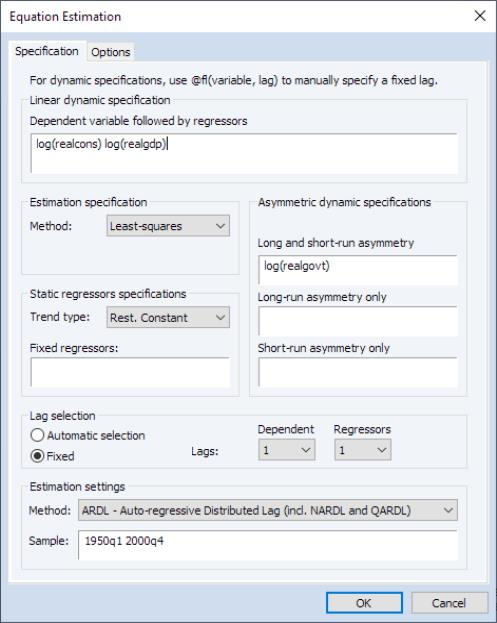and click on OK to estimate the new specification.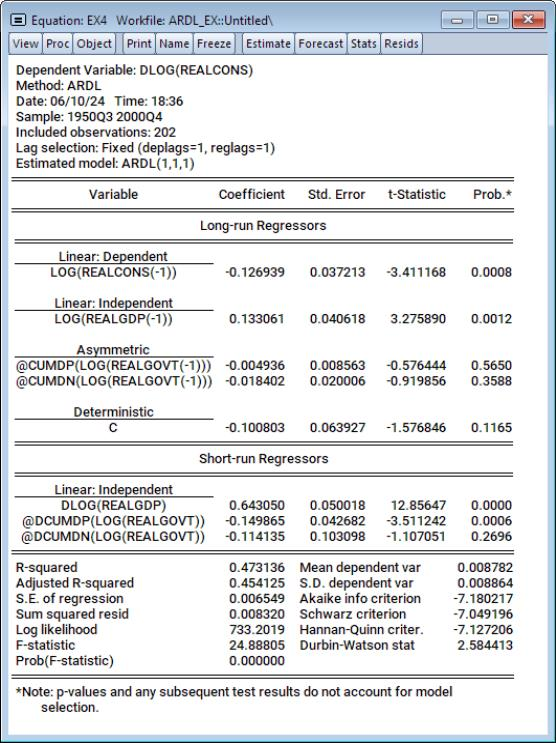Notice that the LOG(REALGOVT) is now split into four variables corresponding to the positive and negative cumulative sums and cumulative difference sums using the labels “@CUMDP(LOG(REALGOVT(-1))” and “@CUMDN(LOG(REALGOVT(-1))” for the long-term effects, and the labels “@DCUMDP(LOG(REALGOVT))” and “@DCUMDN(LOG(REALGOVT))” for the short-term effects.
It is extremely important to note that in contrast to purely symmetric ARDL models which display the ITD representation Equation (29.1) results as the default output (with an option to display the results for the CEC equation Equation (29.8) using the Conditional Error Correction (Long Run) Form view), the default output for asymmetric NARDL models shows the CEC equation Equation (29.18) representation. This difference in the default display is due to the need to support NARDL partial asymmetry models which may only be specified in the context of a CEC model.
We may also test whether the asymmetric assumptions for LOG(REALGOVT) are valid by testing for symmetry. Click on View/ARDL Diagnostics/Symmetry Test to conduct the test: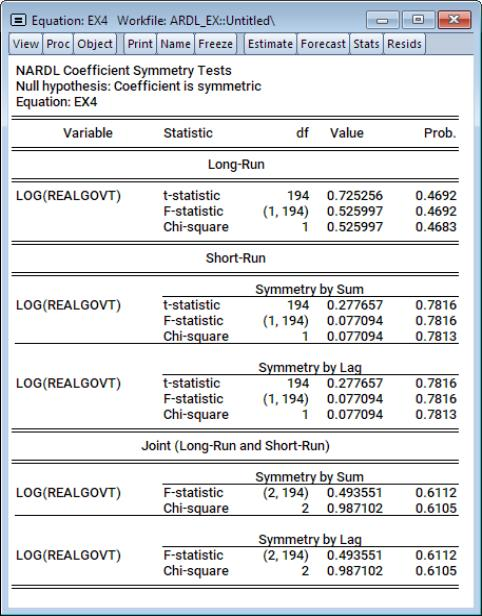EViews displays a table of test results for long-run and short-run restrictions. In this case, we do not reject the null hypothesis of dual asymmetry against the long-run or short-run partial asymmetric alternative.
We may also display the cumulative dynamic multiplier curves. Click on View/ARDL Diagnostics/Dynamic Multiplier Graph...} to bring up the dynamic multiplier dialog: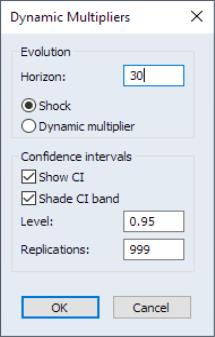Note that confidence interval settings are now available in the dialog for our asymmetric NARDL model since dynamic multiplier CIs are derived for the absolute difference in paths resulting from the positive and negative asymmetric components of a given regressor. You may check the Show CI to display the CIs, and Shade CI band to display the CIs as bands instead of lines. The Level edit field controls the size of the CI, and the Replications governs how many replications to use in resampling for computing the CI.
To proceed with our example, enter “30” as the horizon length and leave everything at else at the default values. Click on OK to continue.
The results are displayed in a spool, with one graph for each of the distributed lag explanatory variables. The first node shows the CDM for LOG(REALGDP) on the dependent variable LOG(REALCONS).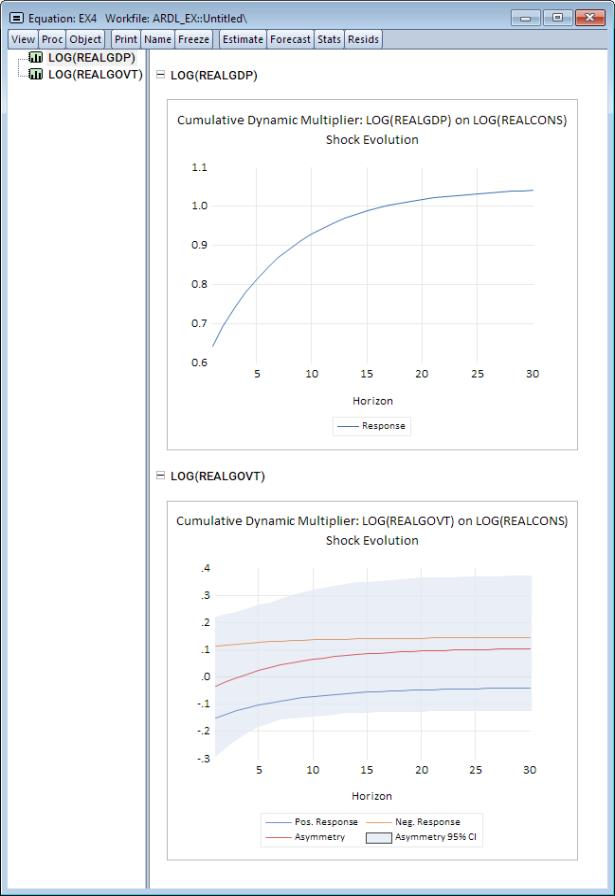Notice that the path approaches the long-run value, which is indicated by dashed horizontal line. Since LOG(REALGDP) is symmetric, there is no CI around the CDM.
The second node shows the CDM graph for the asymmetric variable LOG(REALGOVT):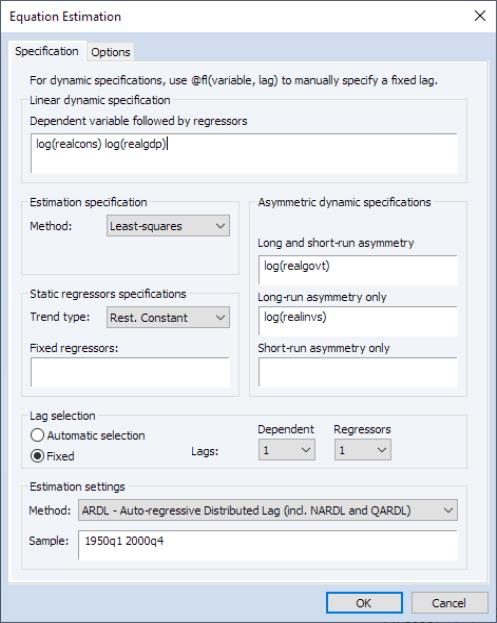Since LOG(REALGOVT) is asymmetric in both the long-run and short-run, we expect the dynamic multiplier curves to differ in both the long-run and short-run. This relationship is seen in the fact that the absolute difference between these paths (the top line with shaded CI interval) never approaches zero. The remaining lines display the CDMs for the positive and negative values, which as expected, approach their long-run values.
Example 5: Asymmetric ARDL(1,1,1,1)
Continuing with the previous example, we add a partially symmetric variable to the list of distributed-lag regressors. We treat real investments (REALINVS) as a variable which is asymmetric in the long-run, but symmetric in the short run. Make a copy of EX4, name the copy EX5, then bring up the estimation dialog by clicking on the Estimate button. Enter “LOG(REALINVS)” in the Long-run asymmetry only edit field, and click on OK to estimate the updated specification.
The results of this estimation are: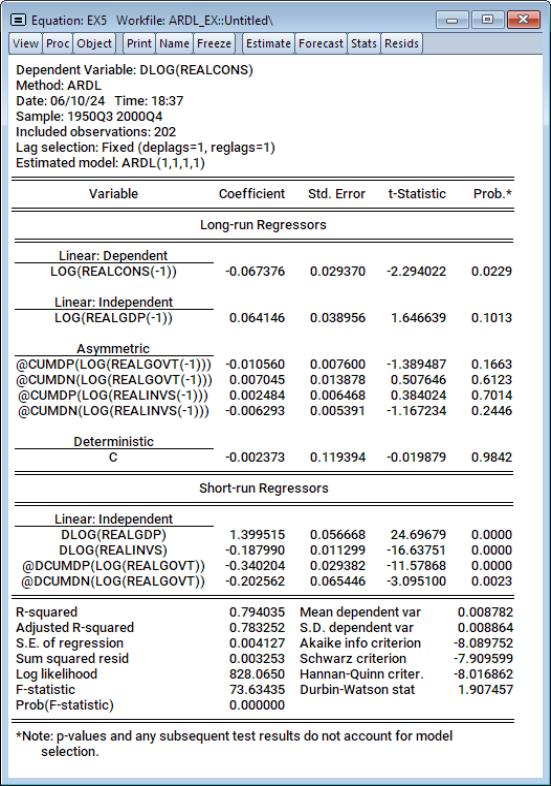Observe now that LOG(REALINVS) is split into its positive and negative cumulative sums in the long-run labeled “@CUMDP(LOG(REALINVS(-1))” and “@CUMDN(LOG(REALINVS(-1))”, and the short-run symmetric effect labeled “@DLOG(REALINVS)”. In contrast, LOG(REALGOVT) is asymmetric in both the long-run and short-run,
We can further study the long-run relationship by clicking on Views/ARDL Diagnostics/Error Correction Results to display the CEC and EC representations,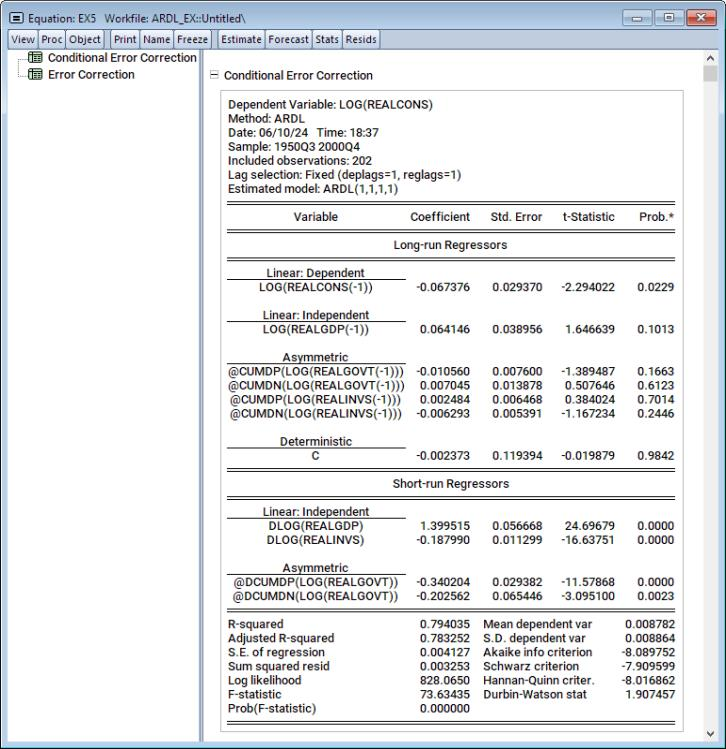and by clicking on Views/ARDL Diagnostics/Cointegrating Relation to display the equilibrium relationship,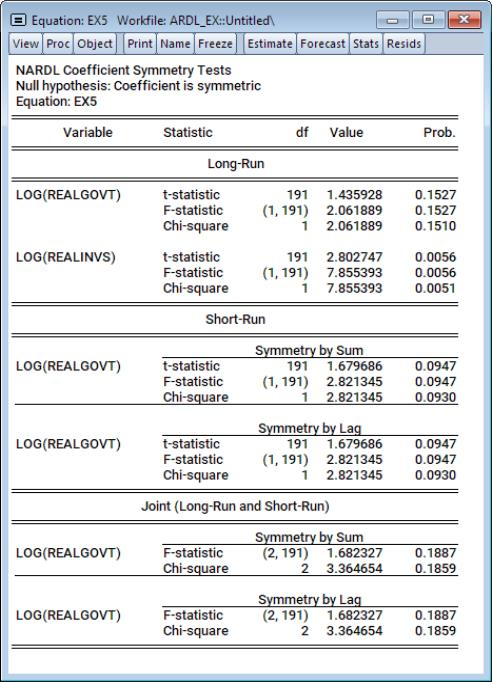We see here that both LOG(REALGOVT) and LOG(REALINVS) enter the cointegrating relationship asymmetrically, while LOG(REALGDP) enters symmetrically.
Notice also the full expression for the error correction relationship in the Cointegrating Specification table. It is worth pointing out that the expression uses “@CUMDP” and “@CUMDN” functions that contain the series of interest and an initialization date. This is the actual, working specification for these expressions required for evaluation. For brevity, the initialization argument does not appear in the labels used in the Cointegrating Coefficients table results.
We can also conduct a symmetry test by selecting the ARDL Diagnostics/Symmetry Test menu item: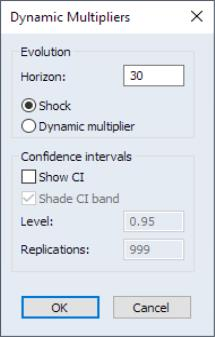Since LOG(REALGOVT) is a fully asymmetric variable, it is tested for symmetry along both the long-run and short-run dimensions, while LOG(REALINVS) is estimated and tested only in the long-run dimension. We fail to reject symmetry for LOG(REALGOVT) at conventional significance levels in both the long-run and short-run but reject the null of LOG(REALINVS) long-run symmetry at conventional sizes.
To display the dynamic multiplier curves we again click on View/ARDL Diagnostics/Dynamic Multiplier Graph... to bring up the dynamic multiplier dialog: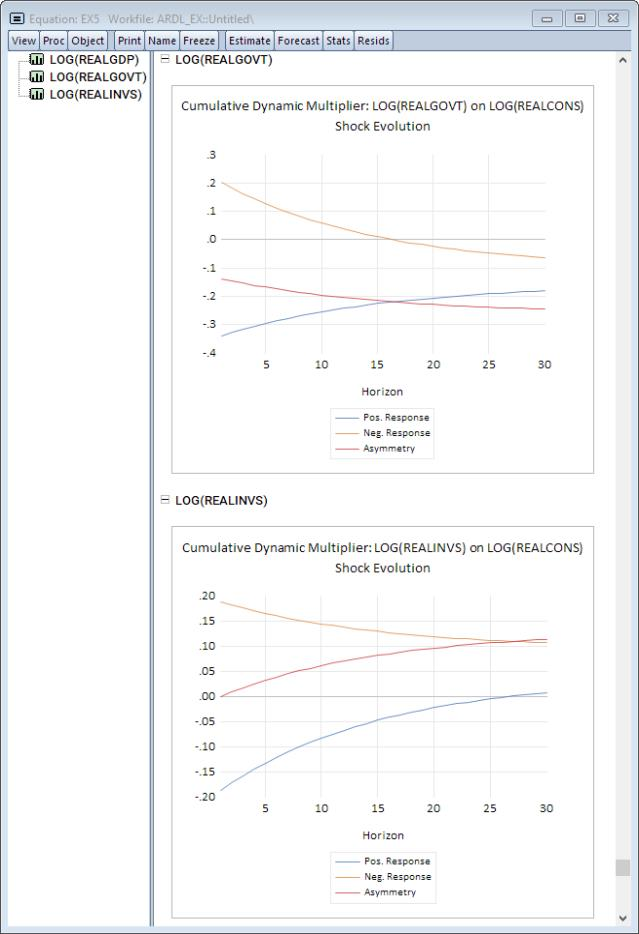Here we will generate the curves for 30 periods without displaying confidence intervals. As before, enter “30” as the horizon length, deselect the Show CI checkbox and click on OK to proceed. EViews will display a spool containing CDM graphs for each of the explanatory variables. Of particular interest here is the final curve associated with LOG(REALINVS):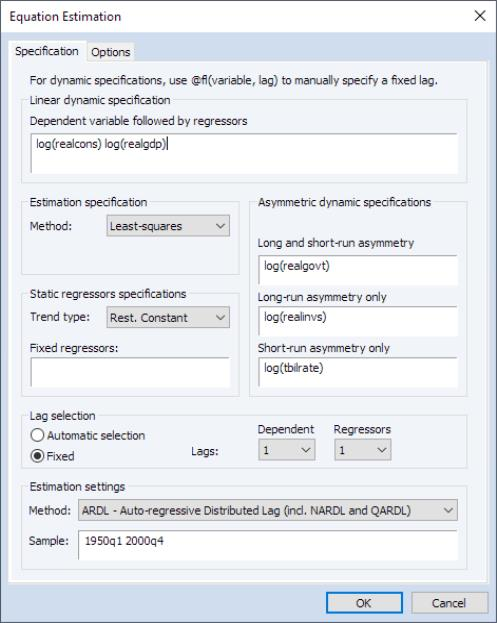Recall that the LOG(REALINVS) is asymmetric in the long-run, but is symmetric in the short-run. This dynamic behavior is clearly seen by noting the topmost absolute asymmetry curve starts off at zero, then diverges in the long-run.
Example 6: Asymmetric ARDL(1,1,1,1,1)
Next, we add a partially symmetric variable to the list of distributed-lag regressors. In particular, we will treat the log of the t-bill rate, LOG(TBILRATE), as a variable which is asymmetric in the short-run, but symmetric in the long-run. Copy EX5, name the copy EX6, then bring up the estimation dialog by clicking on the Estimate button. Enter “LOG(TBILRATE)” in the Short-run asymmetry only edit field, then click on OK to estimate the updated equation. EViews displays the CEC results: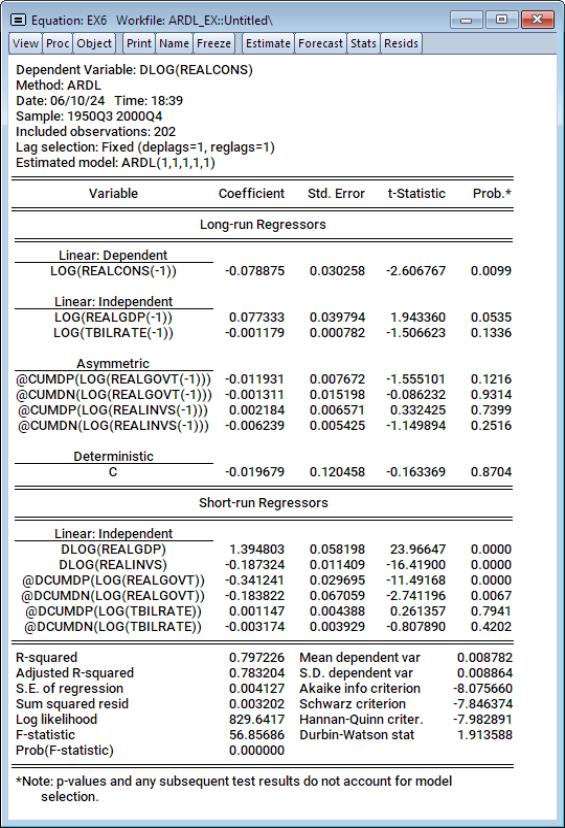LOG(TBILRATE) appears as a symmetric long-run variable through the variable labeled “LOG(TBILRATE(-1))”, and asymmetrically in the short-run through the variables labeled “@DCUMDP(LOG(TBILRATE))” and @DCUMDN(LOG(TBILARATE))”.
Lastly, we display the dynamic multipliers for this specification. Click on View/ARDL Diagnostics/Dynamic Multiplier Graph... to bring up the dynamic multiplier dialog. As before, enter “30” as the horizon length and deselect the Show CI checkbox. Click on OK to continue: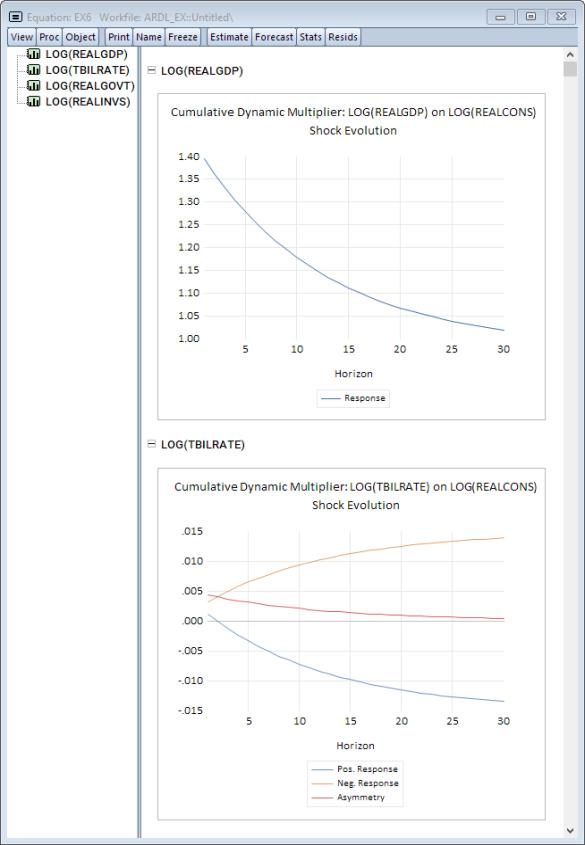As in the previous example, our focus is on the final graph. LOG(TBILRATE) is asymmetric in the short-run, but symmetric in the long-run. Accordingly, the absolute asymmetry graph on the top is above zero at the start of the evolution, but then settles to zero as we approach the long-run.